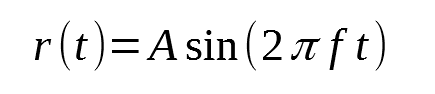# Sine-wave acceleration problem

Hi,

I’m trying to make my rigidbody float above the ground in a sinusoidal fashion. What’s important, is that I don’t want to move it directly via transform or velocity, but by applying a continuous, changing force.
Funny thing is - it actually works correctly in both of the aforementioned cases, but not in the desired one.

The results are not-that-bad - the movement is smooth and all, but - first of all - it’s way too subtle. Secondly, the preset amplitude and frequency seem not to affect it the way they should.

A word about implementation. I’ve followed the sine wave equation, starting at:where

• A - amplitude
• f - frequency
• t - time; in code get’s replaced by Time.time

Now, since I want AddForce to do the dirty work, I need to find the acceleration formula. So, I’m finding the second derivative (with respect to t) of the first equation:

So it’s almost set.

However, I do realize that in Unity I cannot affect the acceleration (or force) by directly setting its level - all I can do is add some in a certain direction.

That’s exactly how I went about it:

``````var difference = newAccel - lastAccel;
``````

Finally, here’s the complete code:

``````using System.Collections;
using System.Collections.Generic;
using UnityEngine;

public class Forcer : MonoBehaviour {

[SerializeField]
float _frequency;
float Frequency { get { return _frequency; } set { _frequency = value; } }

[SerializeField]
float _amplitude;
float Amplitude { get { return _amplitude; } set { _amplitude = value; } }

Rigidbody ThisBody { get; set; }

Vector3 LastSetAcceleration{ get; set; }

void Awake()
{
ThisBody = GetComponent<Rigidbody>();
}
void Start()
{
LastSetAcceleration = Vector3.zero;
}

void OnEnable()
{
ThisBody.useGravity = false;
}
void OnDisable()
{
ThisBody.useGravity = true;
}

void FixedUpdate()
{
var desiredAccelerationValue = -4 * Amplitude * Mathf.Pow(Mathf.PI, 2) * Mathf.Pow(Frequency, 2) * Mathf.Sin(2 * Mathf.PI * Frequency * Time.fixedTime);
var desiredAcceleration = desiredAccelerationValue * Vector3.up;

var difference = desiredAcceleration - LastSetAcceleration;

LastSetAcceleration = desiredAcceleration;
}
}
``````

NOTE: UseGravity is disabled by default, the code implementation is redundant at the moment.

Summing up - my question is: why on earth do velocity and disposition equations work whereas adding acceleration results in some bizarre behaviour? Where do I make that crucial mistake?

All help’s appreciated! Thanks in advanceI know it’s an old question, but i still had your question open (besides 500 others).

Well, your main issue is this:

``````var difference = desiredAcceleration - LastSetAcceleration;